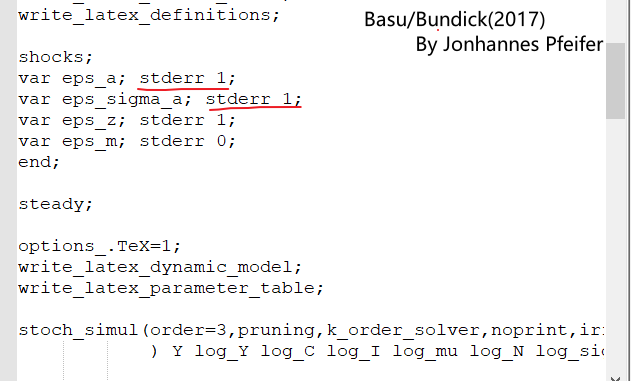# Tariff volatility shocks in SOE don't get to steady state

Hello everyone:
I try to add tariff uncertainty to nonlinear small open economy model.
Although the code can run, but the result is obviously wrong.
(The model based on Gali Chapter 8 third order)

(2)the impluse response graph of Y, C is up to 10^5. the variable value of “variables_irfss” is big.
I don’t know why the results is so big.

1. You did not provide the `sss`-function.
2. The underlying level shock has a variance of 1, i.e. a 1 standard deviation shock doubles tau from 1 to 2. That causes the large numbers.

Thanks,jpfeifer.
I see you had replicated Basu/Bundick (2017): Uncertainty shocks in a model of effective demand", all level shock has a 1 standard deviation shock as following，other economists also set the standara deviation of the level shock to 1.I think the level shocks should: var eps_sigma_a; stderr 0.002; var eps_a; stderr 0.01; I don’t understand why stderr set to 1.

Yes, but their process is

``````[name='Preference shock process level equation']
a=(1-rho_a)*a_bar+rho_a*a(-1)+sigma_a(-1)*eps_a;
[name='Preference shock volatility process']
sigma_a=(1-rho_sigma_a)*sigma_a_bar+rho_sigma_a*sigma_a(-1)+sigma_sigma_a*eps_sigma_a;
``````

so that the shock term has standard deviation `sigma_a_bar` with `sigma_a_bar =0.0026251;`

You have `sigma_tau_bar=1;`